# Numerical investigation of stagnation point flow over a stretching sheet with convective boundary conditions

## Abstract

In this study, the mathematical modeling for stagnation point flow over a stretching surface with convective boundary conditions is considered. The transformed boundary layer equations are solved numerically using the shooting method. Numerical solutions are obtained for the skin friction coefficient, the surface temperature as well as the velocity profiles. The features of the flow and heat transfer characteristics for various values of the Prandtl number, stretching parameter and conjugate parameter are analyzed and discussed.

## 1 Introduction

Problems related to convection boundary layer flows are important in engineering and industrial activities. Such flows are applied to manage thermal effects in many industrial outputs, for example, in electronic devices, computer power supply and also in an engine cooling system such as a heatsink in a car radiator. Sakiadis  was the first to study the boundary layer flow on a continuous solid surface moving at a constant speed. Due to entrainment of the ambient fluid, this boundary layer flow is quite different from the Blasius flow past a flat plate. Sakiadis’s theoretical predictions for Newtonian fluids were later corroborated experimentally by Tsou et al. . Flow of a viscous fluid past a stretching sheet is a classical problem in fluid dynamics. Crane  was the first to study the convection boundary layer flow over a stretching sheet. The heat and mass transfer on a stretching sheet with suction or blowing was investigated by Gupta and Gupta . They considered an isothermal moving plate and obtained the temperature and concentration distributions. Chen and Char  studied the laminar boundary layer flow and heat transfer from a linearly stretching, continuous sheet subjected to suction or blowing with prescribed wall temperature and heat flux. Stagnation flow towards a shrinking sheet was then investigated by Wang  who considered the prescribed wall temperature case. Ishak et al.  studied the MHD stagnation point flow towards a stretching sheet, mixed convection towards a vertical and continuosly stretching sheet and post stagnation-point towards a vertical and linearly stretching sheet. This type of problem was then extended to viscous fluids, viscoelastic fluids or micropolar fluids by many investigators by considering the usually applied boundary conditions, either prescribed wall temperature or prescribed wall heat flux. Recently, Mohamed et al.  studied the stagnation point flow over a stretching sheet and Hayat et al.  investigated the flow of a second grade fluid over a stretching surface with Newtonian heating.

On the other hand, Merkin  has shown that in general, there are four common heating processes specifying the wall-to-ambient temperature distributions, namely (i) constant or prescribed wall temperature; (ii) constant or prescribed surface heat flux; (iii) Newtonian heating (NH); and (iv) convective/conjugate boundary conditions (CBC), where heat is supplied through a bounding surface of finite thickness and finite heat capacity. The interface temperature is not known a priori but depends on the intrinsic properties of the system, namely the thermal conductivity of the fluid or solid. Recent demands in heat transfer engineering have requested researchers to develop various new types of heat transfer equipments with superior performance, especially compact and light-weight ones. With the increasing need for small-size units, focus has been cast on the effects of the interaction between developments of thermal boundary layers in both fluid streams and of axial wall conduction, which usually affects the heat exchanges performance. Since the early paper by Luikov et al. , many contributions to the topic of conjugate heat transfer have been made. The conjugate/convective boundary condition has been used only quite recently by Aziz  who studied the laminar thermal boundary layer over a flat plate. This Blasius flow with the conjugate boundary condition then has been revisited by Rashidi and Erfani  and Magyari . Makinde and Aziz  considered the hydromagnetic heat and mass transfer over a vertical plate. Ishak et al. [18, 19] have studied the thermal boundary layer flow on a moving plate (Sakiadis flow) with radiation effects. Recently, Merkin and Pop , Yao et al. , Yacob et al.  and Yacob and Ishak  investigated the boundary layer flow past a shrinking/stretching sheet with convective boundary conditions in a viscous fluid, nanofluid or micropolar fluid, respectively. Excellent reviews of the topics of convective heat transfer problems can be found in the books by Kimura et al.  and Martynenko and Khramtsov .

Motivated by the works of Wang  and Yacob and Ishak , we aim in this study to investigate the problem of stagnation point flow over a stretching sheet with convective boundary conditions. The governing nonlinear partial differential equations are first transformed into a system of ordinary differential equations by a similarity transformation before being solved numerically using the shooting method (see Salleh et al.  for more details about this method).

## 2 Mathematical formulation

A steady two-dimensional stagnation-point flow over a stretching/shrinking plate immersed in an incompressible viscous fluid of ambient temperature ${T}_{\mathrm{\infty }}$ is considered. It is assumed that the external velocity ${u}_{e}\left(x\right)$ and the stretching velocity ${u}_{w}\left(x\right)$ are of the forms ${u}_{e}\left(x\right)=ax$ and ${u}_{w}\left(x\right)=bx$, where a and b are constants. The physical model and coordinate system of this problem are shown in Figure 1. It is further assumed that the plate is subjected to a conjugate boundary condition. The boundary layer equations are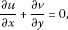(1)(2)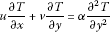(3)

subject to the boundary conditions (Salleh et al.  and Aziz )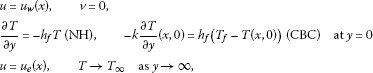(4)

where u and v are the velocity components along the x and y directions, respectively. Further, T is temperature, ${T}_{f}$ is the temperature of the hot fluid, ν is the kinematic viscosity, k is the thermal conductivity, α is the thermal diffusivity and ${h}_{f}$ is the heat transfer coefficient.

We now introduce the following similarity variables (see Salleh et al.  and Aziz ):

(5)

where ψ is the stream function defined as $u=\frac{\partial \psi }{\partial y}$ and $v=-\frac{\partial \psi }{\partial x}$, which identically satisfies Equation (1). Thus, we have

$u=ax{f}^{\prime }\left(\eta \right),\phantom{\rule{2em}{0ex}}v=-{\left(a\nu \right)}^{1/2}f\left(\eta \right),$
(6)

where prime denotes differentiation with respect to η. Substituting (5) and (6) into Equations (2) and (3), we obtain the following nonlinear ordinary differential equations:(7)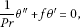(8)

where $\mathit{Pr}=\frac{\nu }{\alpha }$ is the Prandtl number. The boundary conditions (4) become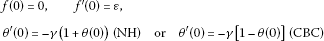(9)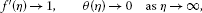(10)

where $\epsilon =\frac{b}{a}\ge 0$ is the stretching parameter. Further, $\gamma ={h}_{f}{\left(\frac{\nu }{a}\right)}^{1/2}$ (NH) or $\gamma ={h}_{f}{\left(\frac{\nu }{a}\right)}^{1/2}{k}^{-1}$ (CBC) is the conjugate parameter for the convective boundary condition. It is noticed that $\gamma =0$ is for the insulated plate and $\gamma \to \mathrm{\infty }$ is when the surface temperature is prescribed. The physical quantities of interest are the skin friction coefficient ${C}_{f}$ and the local Nusselt number ${\mathit{Nu}}_{x}$ which are given by

${C}_{f}=\frac{{\tau }_{w}}{\rho {u}_{e}^{2}},\phantom{\rule{2em}{0ex}}{\mathit{Nu}}_{x}=\frac{x{q}_{w}}{k\left({T}_{w}-{T}_{\mathrm{\infty }}\right)},$
(11)

where ρ is the fluid density. The surface shear stress ${\tau }_{w}$ and the surface heat flux ${q}_{w}$ are given by

${\tau }_{w}=\mu {\left(\frac{\partial u}{\partial y}\right)}_{y=0},\phantom{\rule{2em}{0ex}}{q}_{w}=-k{\left(\frac{\partial T}{\partial y}\right)}_{y=0}$
(12)

with $\mu =\rho \nu$ and k being the dynamic viscosity and the thermal conductivity, respectively. Using the similarity variables in (5) gives

(13)

where ${\mathit{Re}}_{x}=\frac{{u}_{e}x}{\nu }$ is the local Reynolds number and ${\mathit{Nu}}_{x}$ is the local Nusselt number.

## 3 Numerical method

The system of boundary value problem (BVP) (7)-(10) was solved numerically via the shooting technique  by converting it into an equivalent initial value problem (IVP). In this technique, we choose a suitable finite value of ${\eta }_{\mathrm{\infty }}$ (where ${\eta }_{\mathrm{\infty }}$ corresponds to $\eta \to \mathrm{\infty }$) which depends on the values of the parameters considered. First, the system of equations (7) and (8) is reduced to a first-order system (by introducing new variables) as follows: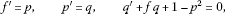(14)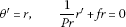(15)

with the boundary conditions

(16)

Now, we have a set of ‘partial’ initial conditions

The Runge-Kutta-Fehlberg method will be adopted to solve the applicable initial value problem. In order to integrate Equations (14) and (15) as an IVP, we require a value for ${f}^{″}\left(0\right)$ and $\theta \left(0\right)$, i.e., ${\alpha }_{1}$ and ${\alpha }_{2}$ respectively. Since these values are not given in the boundary conditions (16), suitable guess values for ${f}^{″}\left(0\right)$ and $\theta \left(0\right)$ are made and integration is carried out. Then, we compare the calculated values for ${f}^{\prime }\left(\eta \right)$ and $\theta \left(\eta \right)$ at ${\eta }_{\mathrm{\infty }}$ with the given boundary conditions ${f}^{\prime }\left({\eta }_{\mathrm{\infty }}\right)=1$ and $\theta \left({\eta }_{\mathrm{\infty }}\right)=0$ respectively and adjust the estimated values of ${f}^{″}\left(0\right)$, $\theta \left(0\right)$ and ${\eta }_{\mathrm{\infty }}$ to give a better approximation for the solution. This computation is done with the aid of shootlib file in Maple software. In this study, the boundary layer thickness ${\eta }_{\mathrm{\infty }}$ between 2 and 8 was used in the computation, depending on the values of the parameters considered so that the boundary condition at ‘infinity’ is achieved.

## 4 Results and discussion

Equations (7) and (8) subject to the boundary conditions (9) and (10) were solved numerically using the shooting method with three parameters considered, namely the Prandtl number Pr, the conjugate parameter γ and the stretching parameter ε. From the numerical solution, it is known that the boundary layer thicknesses ${\eta }_{\mathrm{\infty }}$ from 2 to 8 is suitable to provide accurate numerical results. Due to the decoupled boundary layer equations (7) and (8), for $\epsilon =0$, it has been found that there is a unique value of the skin friction coefficient, ${f}^{″}\left(0\right)=1.2325877$, which is in very good comparison with the classical value ${f}^{″}\left(0\right)=1.232588$ reported by Hiemenz . Table 1 presents the comparison between the present results with the previously reported results by Wang  and Yacob and Ishak  for various values of the stretching parameter ε. It has been found that they are in good-agreement. We can conclude that this method works efficiently for the present problem, and we are also confident that the results presented here are accurate.

Figure 2 illustrates the variation of the surface temperature $\theta \left(0\right)$ with ε when $\mathit{Pr}=0.72$ and . To get a physically acceptable solution, ε must be greater than or equal to a critical value, say εc, i.e., $\epsilon \ge \epsilon c$. It can be seen from this figure that $\theta \left(0\right)$ bounded to 1 as ε approaches the critical value $\epsilon c\simeq -1.2460$.

Figure 3 shows the variation of the surface temperature $\theta \left(0\right)$ with the Prandtl number Pr, when $\epsilon =1$ and . It is noticed that the increasing value of the Prandtl number caused the decrease of surface temperature $\theta \left(0\right)$. From Figures 2 and 3, the same trend is observed for the variation of the surface temperature, i.e., the surface temperature increases as γ increases.

Figure 4 presents the temperature profiles for various values of Pr. It has been found that as Pr increases, the temperature in the boundary layer decreases, and the thermal boundary layer thickness also decreases. This is because for small values of the Prandtl number, the fluid is highly thermal conductive. Physically, if Pr increases, the thermal diffusivity decreases, and this phenomenon leads to the decreasing of energy ability that reduces the thermal boundary layer.

The temperature profiles with various values of ε are presented in Figure 5, and it has been found again that as ε increases, the temperature decreases, and the thermal boundary layer thickness also decreases, similar to the results presented in Figure 4. The temperature profiles presented in Figure 6 show that the temperature increases as the conjugate parameter increases, in contrast with the results presented in Figures 4 and 5 for the variation of Pr and ε.

Lastly, Figure 7 shows the velocity profiles for different values of ε which produce ${f}^{\prime }\left(0\right)=\epsilon$ and ${f}^{\prime }\left(\eta \right)=1$ as $\eta \to \mathrm{\infty }$. When $\epsilon >1$, the flow has an inverted boundary layer structure and the thickness of the boundary layer decreases with ε. On the other hand, when $\epsilon <1$, the flow has a boundary layer structure, which results from the fact that when $b/a<1$, the external velocity ax of the surface exceeds the velocity bx of the stretching sheet. For this case, the thickness of the boundary layer increases with the increase of ε.

## 5 Conclusion

In this paper, we have theoretically and numerically studied the problem of stagnation point flow over a stretching sheet with the convective boundary condition. It is shown how the Prandtl number Pr, stretching parameter ε and conjugate parameter γ affect the values of the surface temperature $\theta \left(0\right)$ and skin friction coefficient ${f}^{″}\left(0\right)$.

We can conclude that the thermal boundary layer thickness depends strongly on these three parameters. Further, it is seen that an increase in the Prandtl number Pr and stretching parameter ε results in a decrease of the temperature. The reason is that smaller values of Pr are equivalent to increasing thermal conductivity and therefore, heat is capable of diffusing away from the heated wall more rapidly than at higher values of Pr. However, the increase of conjugate parameter γ leads to an increase of the surface temperature $\theta \left(0\right)$.

## References

1. Sakiadis BC: Boundary-layer behavior on continuous solid surfaces: I. Boundary-layer equations for two-dimensional and axisymmetric flow. AIChE J. 1961, 7(1):26-28. 10.1002/aic.690070108

2. Tsou FK, Sparrow EM, Goldstein RJ: Flow and heat transfer in the boundary layer on a continuous moving surface. Int. J. Heat Mass Transf. 1967, 10(2):219-235. 10.1016/0017-9310(67)90100-7

3. Crane LJ: Flow past a stretching plate. Z. Angew. Math. Phys. 1970, 21: 645-647. 10.1007/BF01587695

4. Gupta PS, Gupta AS: Heat and mass transfer on a stretching sheet with suction or blowing. Can. J. Chem. Eng. 1977, 55(6):744-746. 10.1002/cjce.5450550619

5. Chen CK, Char M: Heat transfer on a continuous, stretching surface with suction and blowing. J. Math. Anal. Appl. 1988, 135: 568-580. 10.1016/0022-247X(88)90172-2

6. Wang CY: Stagnation flow towards a shrinking sheet. Int. J. Non-Linear Mech. 2008, 43(5):377-382. 10.1016/j.ijnonlinmec.2007.12.021

7. Ishak A, Nazar R, Pop I: Mixed convection on the stagnation point flow toward a vertical, continuously stretching sheet. J. Heat Transf. 2007, 129(8):1087-1090. 10.1115/1.2737482

8. Ishak A, Nazar R, Pop I: Post stagnation point boundary layer flow and mixed convection heat transfer over a vertical, linearly stretching sheet. Arch. Mech. 2008, 60: 303-322.

9. Ishak A, Jafar K, Nazar R, Pop I: MHD stagnation point flow towards a stretching sheet. Phys. A, Stat. Mech. Appl. 2009, 388(17):3377-3383. 10.1016/j.physa.2009.05.026

10. Mohamed MKA, Salleh MZ, Nazar R, Ishak A: Stagnation point flow over a stretching sheet with Newtonian heating. Sains Malays. 2012, 41(11):1467-1473.

11. Hayat T, Iqbal Z, Mustafa M: Flow of a second grade fluid over a stretching surface with Newtonian heating. J. Mech. 2012, 28(1):209-216. 10.1017/jmech.2012.21

12. Merkin JH: Natural-convection boundary-layer flow on a vertical surface with Newtonian heating. Int. J. Heat Fluid Flow 1994, 15(5):392-398. 10.1016/0142-727X(94)90053-1

13. Luikov AV, Aleksashenko VA, Aleksashenko AA: Analytical methods of solution of conjugated problems in convective heat transfer. Int. J. Heat Mass Transf. 1971, 14(8):1047-1056. 10.1016/0017-9310(71)90203-1

14. Aziz A: A similarity solution for laminar thermal boundary layer over a flat plate with a convective surface boundary condition. Commun. Nonlinear Sci. Numer. Simul. 2009, 14(4):1064-1068. 10.1016/j.cnsns.2008.05.003

15. Rashidi MM, Erfani E: A novel analytical solution of the thermal boundary-layer over a flat plate with a convective surface boundary condition using DTM-Pade. 2009 International Conference on Signal Processing Systems 2009.

16. Magyari E: Comment on ‘A similarity solution for laminar thermal boundary layer over a flat plate with a convective surface boundary condition’ by A. Aziz, Comm. Nonlinear Sci. Numer. Simul. 2009, 14:1064-1068. Commun. Nonlinear Sci. Numer. Simul. 2010, 16: 599-601.

17. Makinde OD, Aziz A: MHD mixed convection from a vertical plate embedded in a porous medium with a convective boundary condition. Int. J. Therm. Sci. 2010, 49(9):1813-1820. 10.1016/j.ijthermalsci.2010.05.015

18. Ishak A: Similarity solutions for flow and heat transfer over a permeable surface with convective boundary condition. Appl. Math. Comput. 2010, 217(2):837-842. 10.1016/j.amc.2010.06.026

19. Ishak A, Yacob N, Bachok N: Radiation effects on the thermal boundary layer flow over a moving plate with convective boundary condition. Meccanica 2011, 46(4):795-801. 10.1007/s11012-010-9338-4

20. Merkin JH, Pop I: The forced convection flow of a uniform stream over a flat surface with a convective surface boundary condition. Commun. Nonlinear Sci. Numer. Simul. 2011, 16(9):3602-3609. 10.1016/j.cnsns.2011.01.007

21. Yao S, Fang T, Zhong Y: Heat transfer of a generalized stretching/shrinking wall problem with convective boundary conditions. Commun. Nonlinear Sci. Numer. Simul. 2011, 16(2):752-760. 10.1016/j.cnsns.2010.05.028

22. Yacob NA, Ishak A, Pop I, Vajravelu K: Boundary layer flow past a stretching/shrinking surface beneath an external uniform shear flow with a convective surface boundary condition in a nanofluid. Nanoscale Res. Lett. 2011, 6(1):1-7.

23. Yacob NA, Ishak A: Stagnation point flow towards a stretching/shrinking sheet in a micropolar fluid with a convective surface boundary condition. Can. J. Chem. Eng. 2012, 90(3):621-626. 10.1002/cjce.20517

24. Kimura S, Kiwata T, Okajima A, Pop I: Conjugate natural convection in porous media. Adv. Water Resour. 1997, 20: 111-126. 10.1016/S0309-1708(96)00025-5

25. Martynenko OG, Khramtsov PP: Free Convective Heat Transfer. Springer, Berlin; 2005.

26. Salleh MZ, Mohamed N, Khairuddin R, Khasi’ie NS, Nazar R: Numerical study of free convection boundary layer flow on a vertical surface with prescribed wall temperature, heat flux and Newtonian heating using shooting method. Proceedings of the International Conference on Software Engineering and Computer Systems (ICSECS’09) UMP, Kuantan 19-21 October 2009, 94-98.

27. Salleh MZ, Nazar R, Pop I: Boundary layer flow and heat transfer over a stretching sheet with Newtonian heating. J. Taiwan Inst. Chem. Eng. 2010, 41(6):651-655. 10.1016/j.jtice.2010.01.013

28. Bailey PB, Shampine LF, Waltman PE: Nonlinear Two Point Boundary Value Problems. Academic Press, New York; 1968.

29. Meade DB, Haran BS, White RE: The shooting technique for the solution of two-point boundary value problems. Maple Technol. 1996, 3: 85-93.

30. Bhattacharyya K, Layek GC: Effects of suction/blowing on steady boundary layer stagnation-point flow and heat transfer towards a shrinking sheet with thermal radiation. Int. J. Heat Mass Transf. 2011, 54: 302-307. 10.1016/j.ijheatmasstransfer.2010.09.043

31. Bhattacharyya K, Mukhopadhyay S, Layek GC: Slip effects on boundary layer stagnation-point flow and heat transfer towards a shrinking sheet. Int. J. Heat Mass Transf. 2011, 54: 308-313. 10.1016/j.ijheatmasstransfer.2010.09.041

32. Ishak A: Thermal boundary layer flow over a stretching sheet in a micropolar fluid with radiation effect. Meccanica 2010, 45: 367-373. 10.1007/s11012-009-9257-4

33. Bachok N, Ishak A, Pop I: Melting heat transfer in boundary layer stagnation-point flow towards a stretching/shrinking sheet. Phys. Lett. A 2010, 374: 4075-4079. 10.1016/j.physleta.2010.08.032

34. Hiemenz K: Die Grenzschicht an einem in den gleichformigen Flussigkeitsstrom eingetauchten geraden Kreiszylinder. Dinglers Polytech. J. 1911, 32: 321-410.

## Acknowledgements

The authors wish to thank the anonymous reviewers for their valuable comments and suggestions. The financial support received from the Universiti Malaysia Pahang (Project Codes: RDU110108 and RDU110390) and the Universiti Kebangsaan Malaysia (Project Code: DIP-2012-31) is gratefully acknowledged.

## Author information

Authors

### Corresponding author

Correspondence to Mohd Zuki Salleh.

### Competing interests

The authors declare that they have no competing interests.

### Authors’ contributions

The authors declare that the study was realized in collaboration with the same engagement.

## Authors’ original submitted files for images

Below are the links to the authors’ original submitted files for images.

## Rights and permissions

Reprints and Permissions

Mohamed, M.K.A., Salleh, M.Z., Nazar, R. et al. Numerical investigation of stagnation point flow over a stretching sheet with convective boundary conditions. Bound Value Probl 2013, 4 (2013). https://doi.org/10.1186/1687-2770-2013-4

• Accepted:

• Published:

• DOI: https://doi.org/10.1186/1687-2770-2013-4

### Keywords

• convective boundary conditions
• mathematical modeling
• stagnation point flow
• stretching sheet